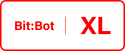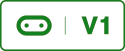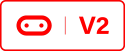# Light Sensitive Robot (Arduino IDE)Go to https://bitbot.l33t.uk/arduino-ide/ultrasonic-example-arduino-ide for instructions on how to configure Arduino IDE for use with the BBC Micro:bit if you haven’t already. This code builds on the code found at https://bitbot.l33t.uk/arduino-ide/porting-bitbot-code-from-micropython-to-c

I have added code for a light seeking robot. I saw little point to create code for a light avoider; the code is similar just inverted. I opened the previous project and created a new tab called _02_LightSensitive and added the below code;

Complete Code Listing
``/* * Light Sensitive algorithm for the 4Tronix Bit:Bot * Author David Bradshaw 2018 */void startLightSensitive(){    uint32_t referenceLevel = getAverageValues();    while (referenceLevel >= 900 )    {        //Bitbot will be stuck in this loop as long as the room is too bright        //The neopixels will be cyan in colour when the room is too bright        setColourRight(0,128,128);         setColourLeft(0,128,128);        referenceLevel = getAverageValues();        Serial.print("REF:   ");        Serial.println(referenceLevel);    }    //When the neopixels turn pink it indicates that the room is dark enough    //and a good reference level has been recorded    neoPixels.clear();    setColourRight(128,0,128);     setColourLeft(128,0,128);    while(1) //infinite loop    {        lightFollow(referenceLevel);    }}void lightFollow(uint32_t referenceLevel){    uint32_t avgBrightness = getAverageValues();  //get average light values    //Follower    if (avgBrightness >= (referenceLevel + 50))  //The difference between ambient light and the follower light must be 50 or more    {        neoPixels.clear();        setColourRight(0,64,0); //Turn the neopixels green to let the user know it can see light        setColourLeft(0,64,0);        int _leftSpeed = (detectLight("LEFT") / 4);  //divide by 4 max val for motors is 255 max val from sensors can be 1023        int _rightSpeed = (detectLight("RIGHT") / 4);        if(_leftSpeed > _rightSpeed)        {            _leftSpeed = 0;        }        else if (_leftSpeed < _rightSpeed)        {            _rightSpeed = 0;        }                move(_leftSpeed, _rightSpeed, 0, 0); //Move left, right or forwards    }    else    {        move(0, 0, 0, 0);        neoPixels.clear();        setColourRight(128,0,128);         setColourLeft(128,0,128);    }}uint32_t getAverageValues(){    //Calculates an ambient light value    uint32_t rightLeftVal = detectLight("LEFT");    uint32_t rightRightVal =detectLight("RIGHT");    return uint32_t((rightLeftVal + rightRightVal)/2);``

I then called the startLightSensitive() method from the loop() method in the _00_programLoop tab.

Colours are the same Cyan = rooms too bright, Pink = waiting for light, Green = following light.

The code for this can be downloaded here Bitbot_Lib_LightSS.zip or the resources section.